# Scattering by free charges LL 2 section 78

• Slides: 15Scattering by free charges LL 2 section 78Incident electromagnetic wave System of charges Energy flux density S Scattered wave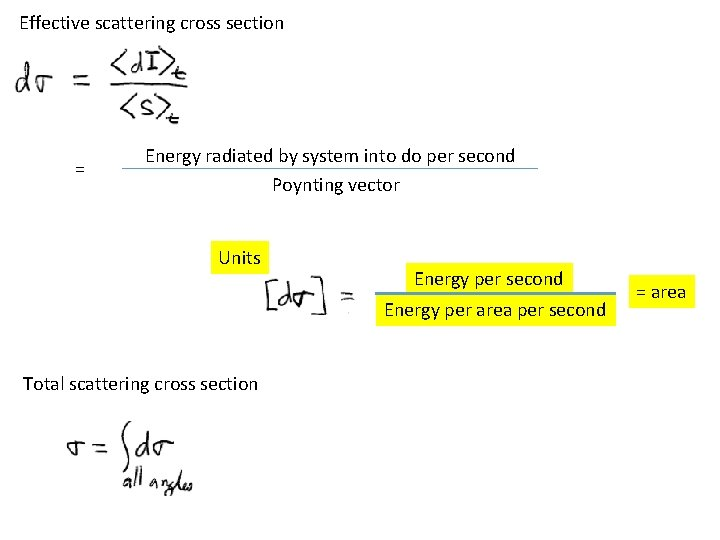Effective scattering cross section = Energy radiated by system into do per second Poynting vector Units Energy per second Energy per area per second Total scattering cross section = area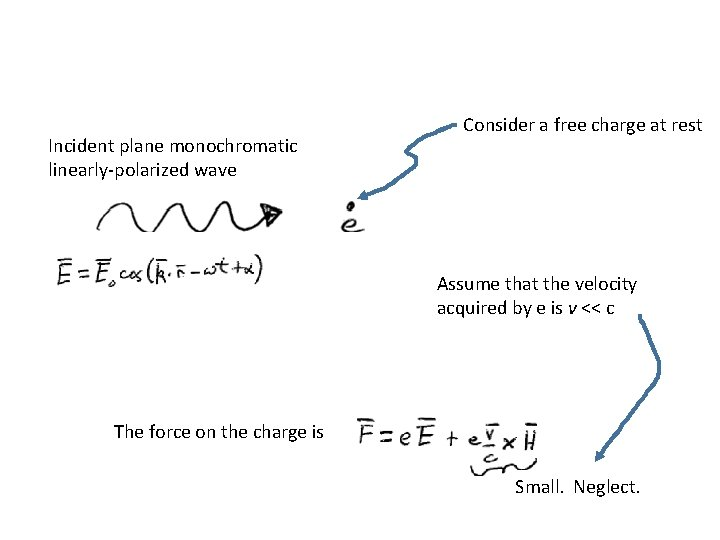Incident plane monochromatic linearly-polarized wave Consider a free charge at rest Assume that the velocity acquired by e is v << c The force on the charge is Small. Neglect.Neglect the effect of displacement. Put the origin at the center of the charge’s vibrations. The field acting on the charge is the same as that at the origin r = 0: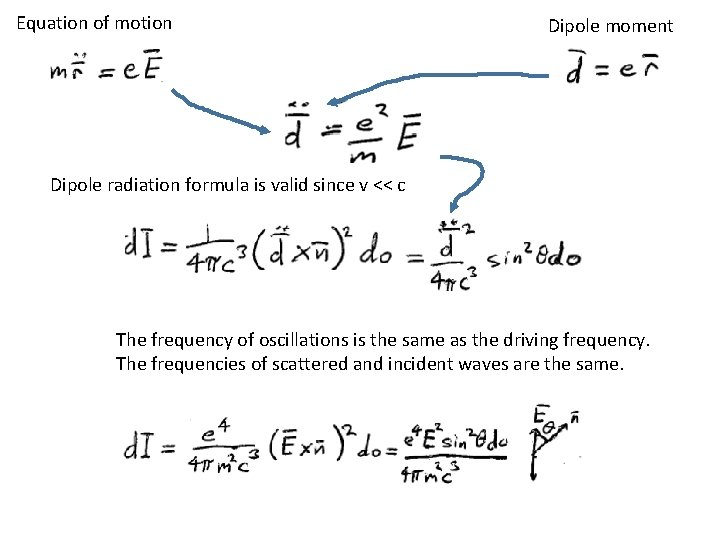Equation of motion Dipole moment Dipole radiation formula is valid since v << c The frequency of oscillations is the same as the driving frequency. The frequencies of scattered and incident waves are the same.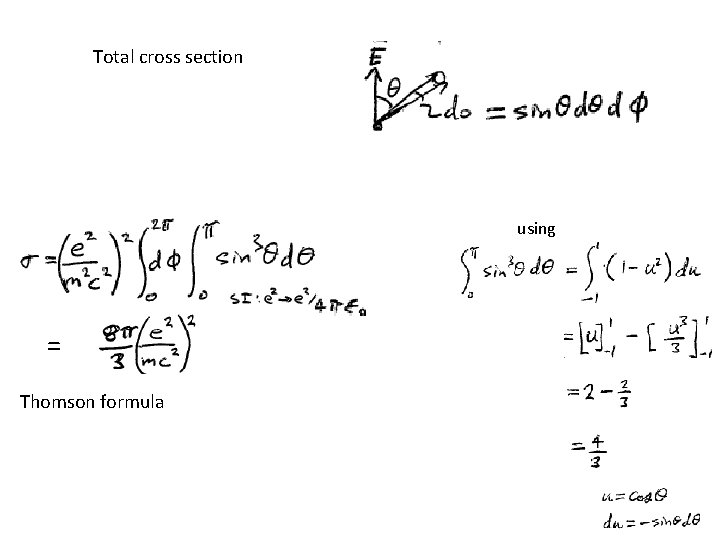Total cross section using = Thomson formulaFor unpolarized light, we need to average over all initial polarization directions. Let e be a unit vector in the direction of E.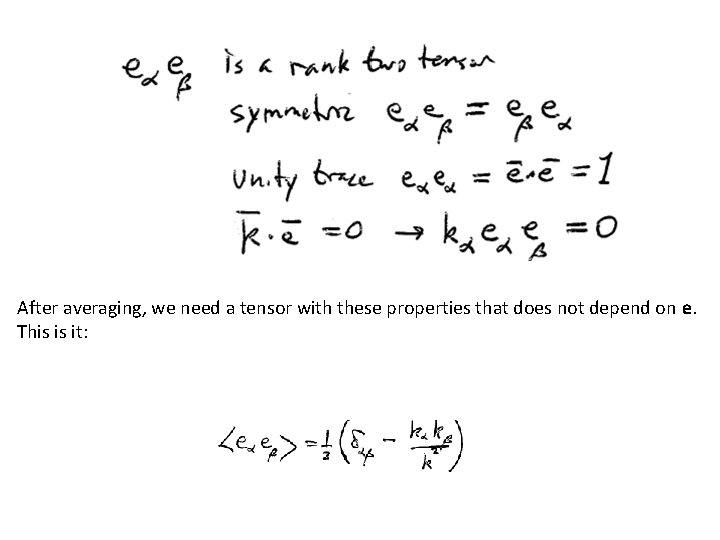After averaging, we need a tensor with these properties that does not depend on e. This is it: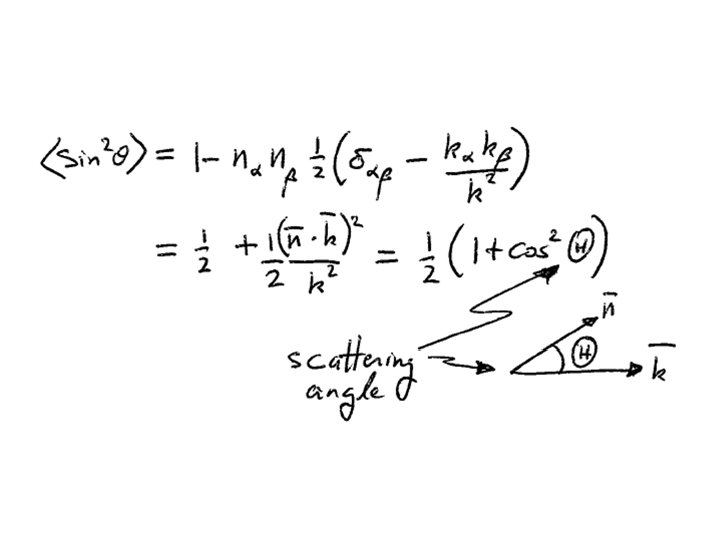Maximum forward and back scattering Half as much for orthogonal scattering. Because there is no radiation along the direction of the electron’s oscillation.Scattering imparts a force on the electron Average energy density in the incident wave = <W> Average energy flux density (energy/area-time) = c <W> Average energy lost to scattering per unit time = c <W> s Average momentum in the incident wave = <W> /c Average momentum per unit time lost to scattering = <W> s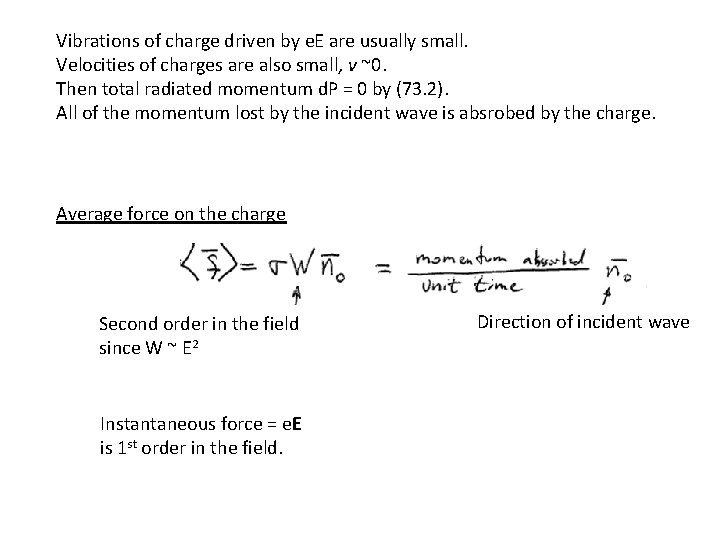Vibrations of charge driven by e. E are usually small. Velocities of charges are also small, v ~0. Then total radiated momentum d. P = 0 by (73. 2). All of the momentum lost by the incident wave is absrobed by the charge. Average force on the charge Second order in the field since W ~ E 2 Instantaneous force = e. E is 1 st order in the field. Direction of incident wave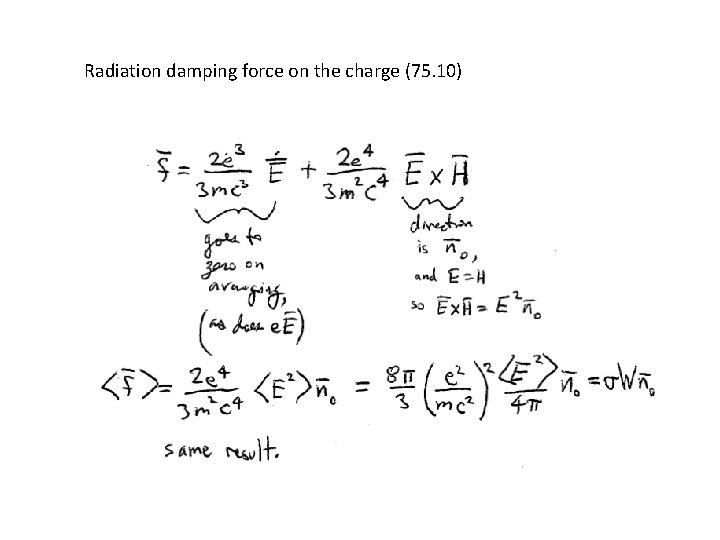Radiation damping force on the charge (75. 10)